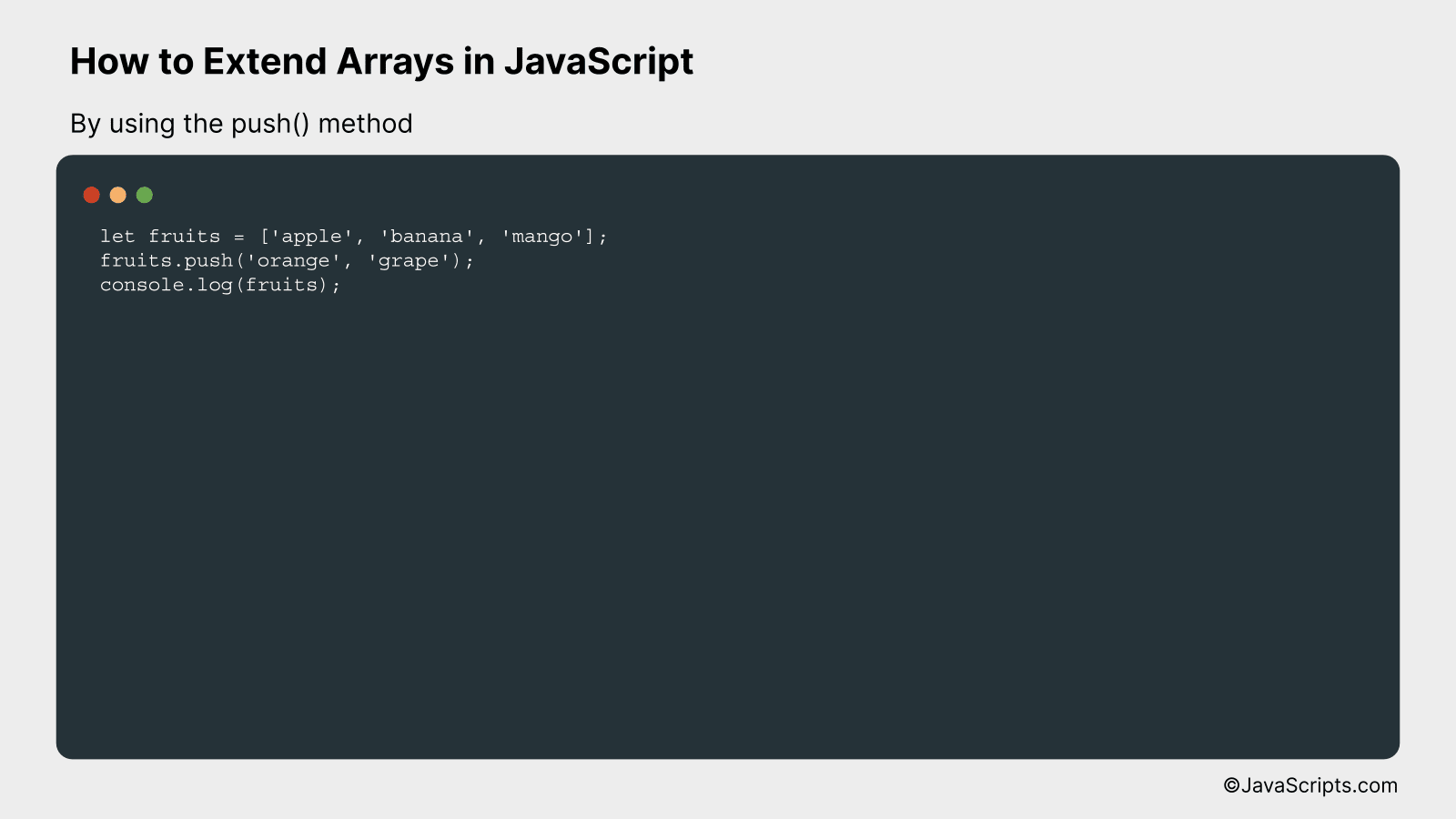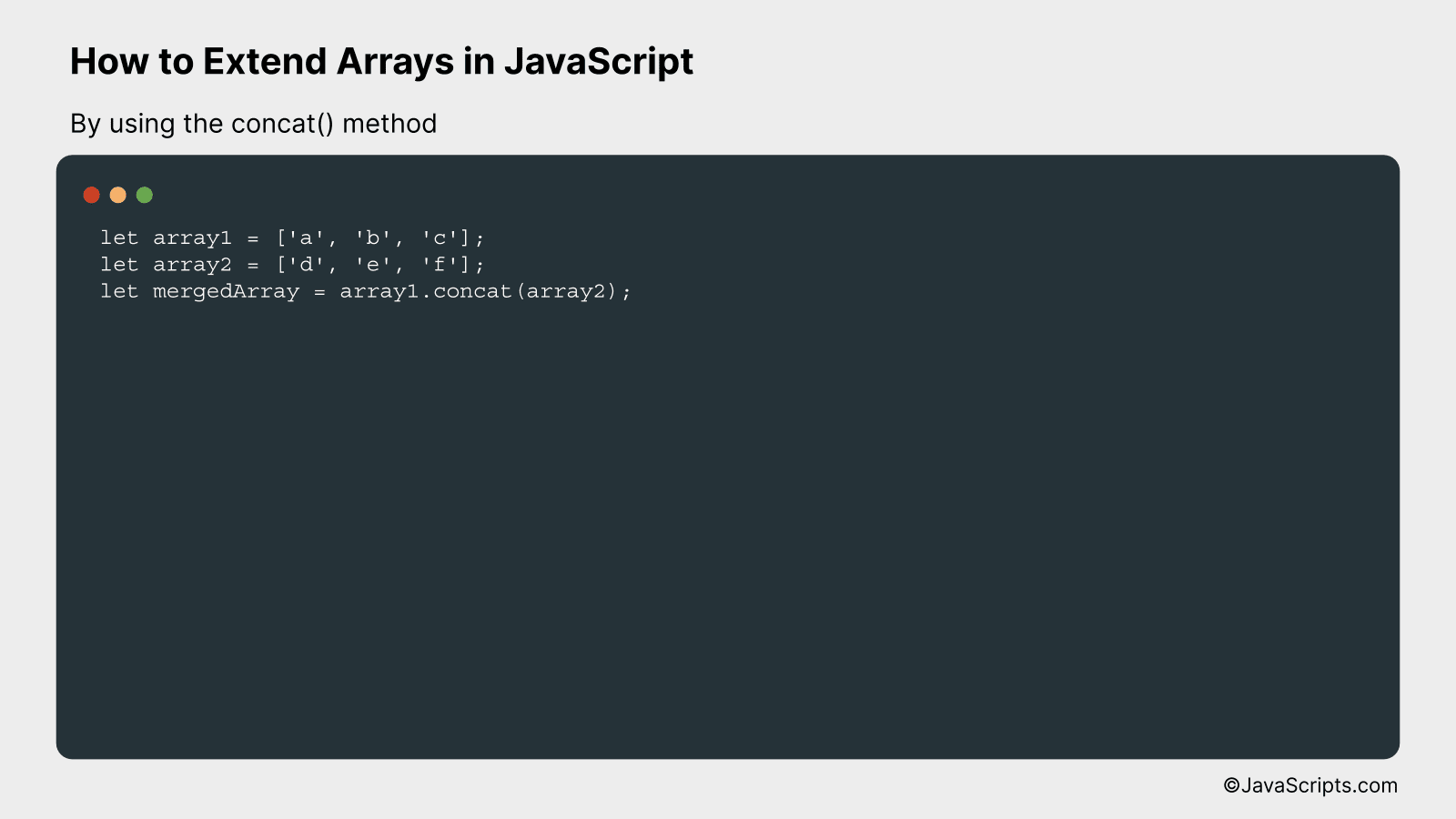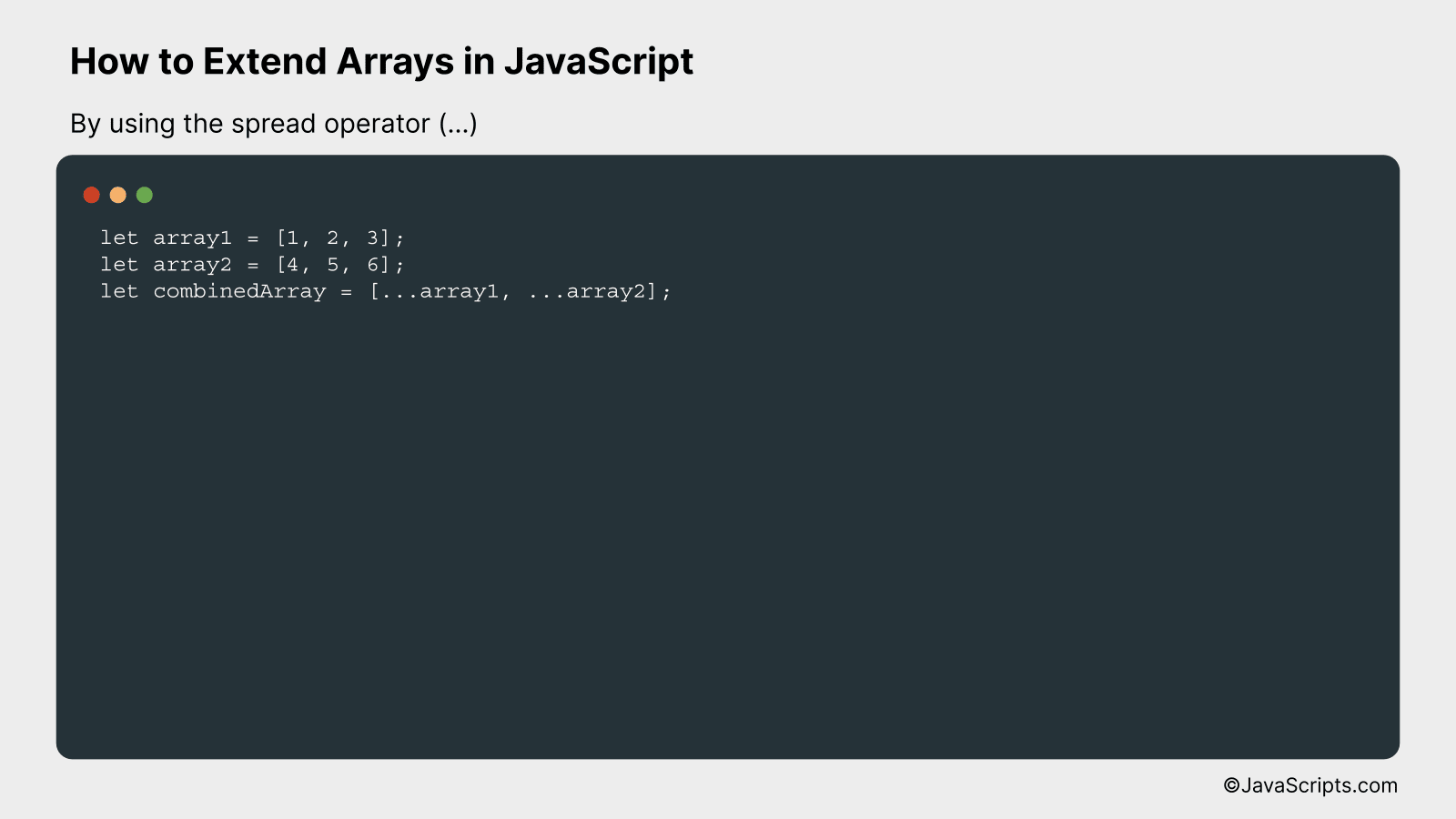# How to Extend Arrays in JavaScript

JavaScript, often known as the backbone of web applications, offers us a myriad of built-in objects to simplify coding, and one such versatile object is the ‘Array’. It’s like a container that allows us to store multiple values in a single variable. But wouldn’t it be more useful if we could extend or append elements to an existing array? Certainly, that’s where we can leverage the power of JavaScript.

You might stumble upon several situations where extending an array might be required. Maybe you’re dealing with a dynamic data set, or appending new items to your shopping cart, or simply combining arrays for data manipulation. Whatever your situation, we’ll walk through various techniques to extend arrays in JavaScript, enhancing your understanding and coding experience.

In this post, we will be looking at the following 3 ways to extend arrays in JavaScript:

• By using the push() method
• By using the concat() method
• By using the spread operator (…)

Let’s explore each…

## #1 – By using the push() methodThe push() method in JavaScript allows you to add one or more elements to the end of an array, extending its length. Understanding this can be easier with a practical example.

``````
let fruits = ['apple', 'banana', 'mango'];
fruits.push('orange', 'grape');
console.log(fruits);
``````

### How it works

The push() method modifies the original array by adding the specified elements to its end and then returns the new length of the array.

• First, we initialize an array ‘fruits’ with three elements: ‘apple’, ‘banana’, ‘mango’.
• Next, we use the push() method to add two more elements: ‘orange’ and ‘grape’ to the end of the ‘fruits’ array.
• Finally, when we log the ‘fruits’ array, it now includes the newly added elements, showing [‘apple’, ‘banana’, ‘mango’, ‘orange’, ‘grape’] as output.

## #2 – By using the concat() methodThe JavaScript concat() method is used to merge two or more arrays and return a new, combined array, without modifying the original arrays. To understand this better, let’s consider an example where we have two arrays and we want to merge them into one.

``````
let array1 = ['a', 'b', 'c'];
let array2 = ['d', 'e', 'f'];
let mergedArray = array1.concat(array2);
``````

### How it works

The concat() method takes one or more arrays as arguments and combines them with the array on which it was called, returning a new array. It doesn’t mutate the original arrays.

• Firstly, we have two arrays, ‘array1’ and ‘array2’, with their respective elements.
• Then, we call the ‘concat()’ method on ‘array1’ and pass ‘array2’ as an argument. This method merges ‘array1’ and ‘array2’.
• The result of this operation, a new array that contains all elements from both ‘array1’ and ‘array2’, is stored in the ‘mergedArray’ variable.
• Thus, ‘mergedArray’ is now [‘a’, ‘b’, ‘c’, ‘d’, ‘e’, ‘f’].

## #3 – By using the spread operator (…)In JavaScript, the spread operator (…) can be used to combine or extend arrays by spreading the elements of one array into another. This operation can be better understood through the following example:

``````
let array1 = [1, 2, 3];
let array2 = [4, 5, 6];
let combinedArray = [...array1, ...array2];
``````

### How it works

With the spread operator, elements of array1 and array2 are extracted or ‘spread’ into the combinedArray. The spread operator is essentially unwrapping the elements of array1 and array2 and placing them directly into combinedArray.

• Step 1: We first declare two arrays, array1 and array2, with some elements.
• Step 2: When declaring the new array, combinedArray, we use the spread operator before array1 and array2. This extracts or ‘spreads’ the individual elements (not the arrays themselves) from array1 and array2.
• Step 3: The spread operator effectively ‘unpacks’ the elements from array1 and array2 and places them into combinedArray. As a result, we get a new array that includes all the elements from both array1 and array2.

Related:

In conclusion, extending arrays in JavaScript is a straightforward and useful skill to master. The methods like ‘push’, ‘unshift’, ‘concat’, and ‘spread operator’ provide flexibility, allowing developers to expand arrays as needed.

Remember, choosing the right method depends on your specific requirements. Practice these techniques and you’ll be proficient in expanding JavaScript arrays in no time.